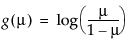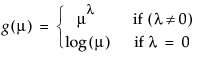Model Response Variable Distribution Default Link Function Traditional Linear Model continuous Normal identity, g(μ) = μ Logistic Regression a count or a binary random variable Binomial logit,Poisson Regression in Log Linear Model a count Poisson log, g(μ) = log(μ) Exponential Regression positive continuous ExponentialLink Function Name Link Function Formula Identity g(μ) = μ LogitProbit g(μ) = Φ-1(μ), where Φ is the standard normal cumulative distribution function Log g(μ) = log(μ) Reciprocal g(μ) =PowerComp LogLog
Variance Functions for Response Distributions lists the variance functions associated with the available distributions for the response variable.
 Distribution Variance Function Normal V(μ) = 1 Binomial V(μ) = μ(1 – μ) Poisson V(μ) = μ Exponential V(μ) = μ2

Help created on 9/19/2017